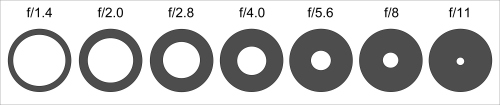Optics

Aperture

The aperture is optical opening in the lens system that determines the amount of light that can pass the lens system. With the aperture stop, normally the iris diaphragm, the opening can be controlled (smaller or larger) and the amount of passing light is defined.

The Aperture Ratio is defined as the ratio between aperture diameter and focal length. The Focal Ratio is the inverse aperture ratio, the ratio between focal length and aperture diameter. This parameter is called the "F-Number" or the "F-Stop". A lower "F-Number" represents more passing light and a higher "F-Number" represents less passing light. Each higher or lower "F-Stop" indicates the half or doubling of light intensity. A f/11 allows only half as much light as a f/8 and a f/4 allows double as much light as a f/8. Typical "F-Stops" are f/2.8, f/4, f/5.6, f/8, f/11, f/16.

Because the maximum aperture is the focal length divided by the diameter of the lens, a lens one inch in diameter with a focal length of 4 inches will allow the same amount of light as a lens three inch in diameter with a focal length of 12 inch:
4 inch / 1 inch = 4    > aperture: f/4
12 inch / 3 inch = 4    > aperture: f/4

Because a telephoto lens is longer (higher focal length), its diameter is will divide more often into the focal length, making the F-Stop higher. So a standard lens with a focal length of 50 often has as the lowest F-Stop a f/2 or even a f/1.4 but a telephoto lens often has as the lowest F-Stop a f/4 or even a f/5.6.typical f-numbers for photo lenses